Monday, 2020-08-03, 3:29 PM

Other pages

# Beer-Lambert law

For a better perspective, it is advisable to read the following sections:

Beer-Lambert law: When light is passed through a medium and comes out at the other side, its intensity will be reduced, because part of the light will be absorbed by the medium. The actual amount of light absorbed by the medium is governed by the Beer-Lambert law. The derivation of the law is as follows:

Lambert’s law: (This law was actually observed by Pierre Bouguer in 1729. Its origin is wrongly attributed to Johann Heinrich Lambert, who only quoted from Bouguer’s work in 1760). This law says that the decrease in intensity 'dI' when light passes through a medium of thickness ‘dx’ is directly proportional to (a) the intensity of the incident radiation ‘I’ and (b) the thickness of the medium. This may be mathematically expressed as:

-dI a I dx        or         -dI = kI dx

where ‘k’ is the proportionality constant. The minus sign indicates that the intensity decreases as light passes through the medium.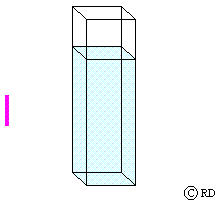Beers Law: (August Beer, 1852): This law says that the decrease in intensity (dI) when light passes through a solution of concentration ‘c’ is directly proportional to the concentration. This may be mathematically expressed as:

-dI a c            or         -dI = kc

Combining the two relationships, we get:

-dI a I c dx     or         -dI = k I c dx               or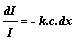Samples in spectroscopy are usually in the form of solutions. The solution of molar concentration ‘c’ is taken in a sample cell. When light passes through the solution in the cell, it has to travel a distance ‘l’ through the solution. Therefore this is called the path length. If ‘I0’ is the intensity of incident light, and it decreases to ‘I’ after passing through the solution, then the intensity is I0 when x=0 and I when x=l. Therefore, integrating the above equation between these limits,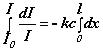or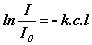or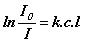oror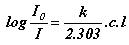If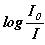is represented as ‘A’ and the constant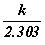as ‘e’, then we can write

A = l                      (Beer-Lambert law)

where ‘A’ is known as the ‘absorbance’ and ‘e’ is a constant for a given substance and called its ‘molar absorptivity coefficient’ (earlier known as ‘molar extinction coefficient’). When the path length is 1 cm and the concentration of the solution is 1 molar, then A = e. Therefore the molar absorptivity coefficient ‘e’ can be defined as the absorbance of a 1 molar solution for a path length of 1 cm. (Therefore most sample cells commercially available have a path length of 1 cm). The unit of ‘e’ is mol-1 cm-1. ‘A’ has no unit since it is a ratio.

If a substance has a very high value for ‘e’, then very low concentrations can be detected and very dilute solutions can be used for spectroscopic analysis. If the value of ‘e’ for the substance is low, then concentrated solutions have to be used for spectroscopic analysis.

Search
Calendar
 «  August 2020  » Su Mo Tu We Th Fr Sa 1 2 3 4 5 6 7 8 9 10 11 12 13 14 15 16 17 18 19 20 21 22 23 24 25 26 27 28 29 30 31
Site friends